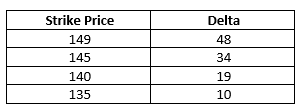Sponsored Content - The option Greeks are often portrayed as a rather esoteric concept, but here I intend to bring the Greeks down to earth and demonstrate their utility for the retail option trader, says Kerry Given of Parkwood Capital, LLC.

The Black Scholes equation computes the theoretical value of an option given the stock or index price, the strike price of the option, the time remaining until option expiration, the current risk-free interest rate (typically the T-bill rate), and the underlying stock or index’s historical volatility. It is an intimidating equation and I won’t scare you with it here. One key point is worthy of emphasis: the probability of the option expiring with value is included in every term of the Black Scholes equation. Thus, probabilities are a key component of an option’s price.

One of the tools mathematicians use to better understand an equation with multiple variables is differential calculus. When we take the derivative of the Black Scholes equation with respect to the stock price, we are holding all of the variables of the equation constant, except the stock price, and computing the effect on the option price of varying the stock price. This derivative of the Black Scholes equation with respect to the stock price is one of the option Greeks, called delta. This derivation reveals a significant approximation. It is unrealistic to assume all of the other variables, such as volatility and time, are fixed while the stock price goes up or down.

The option Greeks are very useful tools although we should not expect precise predictions of future option prices. However, the Greeks are helpful in identifying the primary risk associated with a prospective option trade or identifying a shift in risk as the trade progresses.

While not precisely mathematically correct, many traders use the option Greek, delta, as an estimate of the probability of the option expiring with value. Hence, the Apple (AAPL) Nov (11/19) 145 put with AAPL trading at 149 has a delta of 34, hence a 34% probability of expiring with value and a 66% probability of expiring worthless. If we calculate the probability of this option expiring worthless, we compute 65.4%. Therefore, our use of delta as an approximation for the probability of the option’s value at expiration is reasonably accurate.

The option Greek, delta, may be used in many trading situations. Consider two common trades: selling a naked put and selling a bull put spread. Let’s use AAPL as our example. I am bullish and wish to sell an OTM AAPL put for November expiration. I open the option chain on my broker’s website and scroll down the deltas for the November puts. A brief summary of the choices follows in the table below:A conservative trader may wish to sell the Nov (11/19) 135 put with a delta of 10 since that option has approximately a 90% probability of expiring worthless. Other traders might have a strongly bullish opinion of AAPL’s prospects and choose to sell the Nov (11/19) 145 put with a 66% probability of expiring worthless.

One may also limit the risk of the short put by buying the put one or more strikes below the put we sold, creating a vertical put spread. In either case, I may use the delta approximation for the probability of success as I consider the positioning of the trade.

Delta may also be used to position the stop loss price for a trade. In the example above, the trader sold the Nov (11/19) 135 put with a 90% probability of success. It may be helpful to consider the changing probability of success for the trade as time passes. For example, this trader could decide to stop out the trade if the delta of the short put reaches 35, since the probability of success has declined to about 65%. This procedure for setting the stop loss price could be used for either the naked put or the put spread.

Delta is only one of the option Greeks; future articles will explore how traders use the other option Greeks.

If you want to learn more about real-world trading, absent the marketing hype, check out the private coaching, group classes, and trading services offered by Parkwood Capital. You have entered The No Hype Zone!